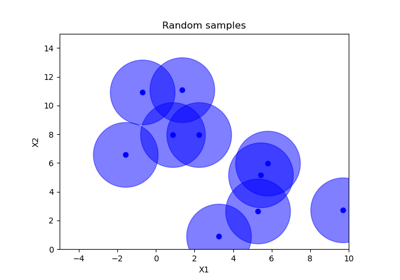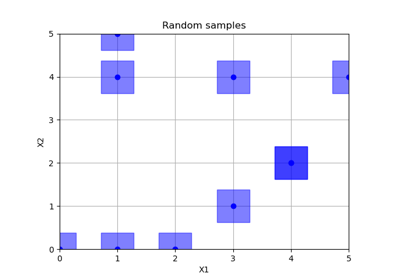# skopt.sampler.Hammersly¶

class skopt.sampler.Hammersly(min_skip=-1, max_skip=-1, primes=None)[source][source]

Creates Hammersley sequence samples. The Hammersley set is equivalent to the Halton sequence, except for one dimension is replaced with a regular grid. It is not recommended to generate a Hammersley sequence with more than 10 dimension.

For dim == 1 the sequence falls back to Van Der Corput sequence.

Parameters
min_skipint, default=-1

minimum skipped seed number. When min_skip != max_skip and both are > -1, a random number is picked.

max_skipint, default=-1

maximum skipped seed number. When min_skip != max_skip and both are > -1, a random number is picked.

primestuple, default=None

The (non-)prime base to calculate values along each axis. If empty, growing prime values starting from 2 will be used.

References

T-T. Wong, W-S. Luk, and P-A. Heng, “Sampling with Hammersley and Halton Points,” Journal of Graphics Tools, vol. 2, no. 2, 1997, pp. 9 - 24.

Methods

 generate(dimensions, n_samples[, random_state]) Creates samples from Hammersly set. set_params(**params) Set the parameters of this initial point generator.
__init__(min_skip=-1, max_skip=-1, primes=None)[source][source]

Initialize self. See help(type(self)) for accurate signature.

generate(dimensions, n_samples, random_state=None)[source][source]

Creates samples from Hammersly set.

Parameters
dimensionslist, shape (n_dims,)

List of search space dimensions. Each search dimension can be defined either as

• a (lower_bound, upper_bound) tuple (for Real or Integer dimensions),

• a (lower_bound, upper_bound, "prior") tuple (for Real dimensions),

• as a list of categories (for Categorical dimensions), or

• an instance of a Dimension object (Real, Integer or Categorical).

n_samplesint

The order of the Hammersley sequence. Defines the number of samples.

random_stateint, RandomState instance, or None (default)

Set random state to something other than None for reproducible results.

Returns
np.array, shape=(n_dim, n_samples)

Hammersley set

set_params(**params)[source]

Set the parameters of this initial point generator.

Parameters
**paramsdict

Generator parameters.

Returns
——-
selfobject

Generator instance.

## Examples using skopt.sampler.Hammersly¶